Quantiacs hosts quantitative trading contests since 2014 and has allocated more than 30M USD to winning algorithms on futures markets. We are expanding the universe of assets you can use and adding new tools.

We offer to users:

• a space to develop code and test ideas for forecasting global financial markets based on Jupyter Notebook and JupyterLab;

• support for Python;

• the power of the full Anaconda data science ecosystem;

• up to free 8 GB of RAM for each trading strategy;

• access to historical data for futures, the Bitcoin future and cryptocurrencies;

• trading strategy templates for getting started;

• prize money. Participate to our competitions and take one of the top spots. Allocations will be made to winners and quants will receive 10% of the generated profits without any downside risk.

Get started with Quantiacs!

• Register to the platform;

• Open the strategy development tab;

• Create a strategy from scratch or clone one of the provided templates; after cloning you will be able to edit your strategy.ipynb file in Jupyter Notebook or JupyterLab;

• Submit strategies and monitor their live performance in your private area. Read carefully the contest page and do not miss the deadline for each contest.

Take a look at the following slides for learning quickly the main steps for taking part to contests (note that, as we are improving the platform constantly and adding new features, the screenshots can be a little bit different from current status):

# Building strategies¶

Our platform allows you to develop trading strategies in a simple and compact way. The trading algorithm should distribute fractions of the available capital (in other words, allocation weights) to the available assets. Our backtester will take care of simulating the performance of the system.

## A basic example for futures¶

The idea is very simple: allocate weights according to the price variation of the asset respect to the day before. If the price variation is positive, the strategy will allocate a positive weight, going long the asset, otherwise it will allocate a negative weight, shorting the asset. This basic example uses liquid futures contracts:

```import qnt.data as qndata
import qnt.stats as qnstats
import qnt.output as output
import qnt.ta as qnta

price_open = futures.sel(field="open")
price_open_one_day_ago = qnta.shift(price_open, periods=1)

strategy = price_open - price_open_one_day_ago

weights = strategy / abs(strategy).sum("asset")

weights = output.clean(weights, futures, "futures")

statistics = qnstats.calc_stat(futures, weights)

output.check(weights, futures, "futures")

output.write(weights)
```

## The detailed steps¶

1. Preparation

We prepare our workspace by importing the needed libraries and loading the data:

```import qnt.data as qndata
import qnt.stats as qnstats
import qnt.output as output
import qnt.ta as qnta

```

futures is an xarray.DataArray structure which contains futures historical data since January 1, 2006. More details on xarray can be found at https://xarray.pydata.org/en/stable/ or User Guide xarray

As the strategy uses price shifts to define weights, we define two auxiliary variables: the open prices of the assets at two consecutive sessions (the second is the open prices of the assts one day ago):

```price_open = futures.sel(field='open')
price_open_one_day_ago = qnta.shift(price_open, periods=1)
```

2. Weight allocation

Quantiacs uses an exposure-based backtester. The trading algorithm should define the fractions of capital which will be distributed to the assets (allocation weights). A positive weight means a long position (buy), a negative value means a short position (sell).

Note that algorithm decisions can use all data available at the close of the session, and will be applied at the opening of the next day's session. The chosen allocation weights are translated to positions (number of contracts to be bought/sold) immediately after the close of the session and transactions are executed at the open of the next day.

This example allocates weights proportionally to the difference between prices for today and yesterday:

```strategy = price_open - price_open_one_day_ago
```

and trades all futures contracts which Quantiacs makes available. Note that we normalize positions so that we are fully invested:

```weights = strategy / abs(strategy).sum('asset')
```

The call to the clean function performs two operations:

1. if there are trading days where the user did not specify any exposure, an exposure of “0” (no allocation) will be used

2. if the total sum of the absolute exposure is larger than 1, normalization to 1 will be applied (i.e. max. allowed leverage is 1).

```weights = output.clean(weights, futures, 'futures')
```

Here is how the weights xarray would look like. Note that for each day we have a weight for each asset, corresponding to how much capital will be distributed to that asset in a long (positive weight) or short (negative weight) position.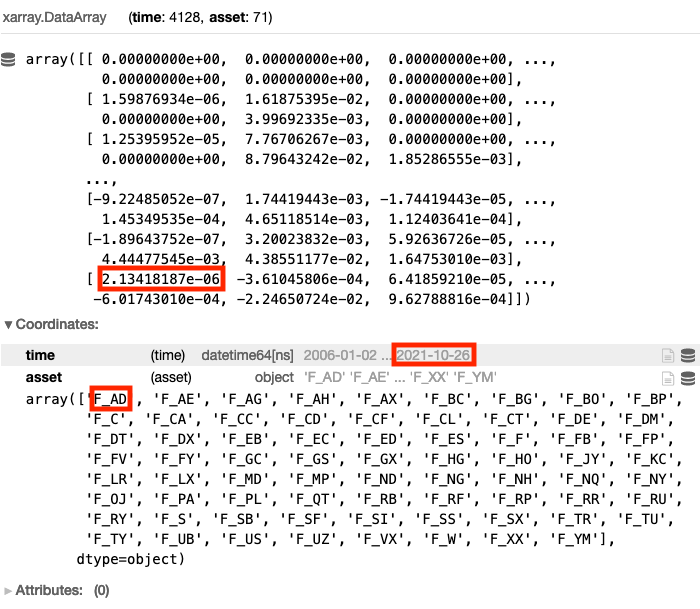In this example we allocated 2.13e-06 to F_AD on 2021-10-26.

We have two coordinates:

• time: the weights are defined for every trading day

• asset: each weight corresponds to a position we would like to make on one particular asset

For an overview of the xarray.DataArray datastructure please consult User Guide xarray

3. Performance estimation

After we have built the algorithm, we can evaluate its performance calculating statistics:

```statistics = qnstats.calc_stat(futures, weights)
```

We can display the values of statistical indicators on a cumulative basis, assuming that we have 1M USD at the starting point:

```display(statistics.to_pandas().tail())
```

The call will produce:

• equity: the cumulative value of profits and losses since inception (1M USD);

• relative_return: the relative daily variation of equity;

• volatility: the volatility of the investment since inception (i.e. the annualized standard deviation of the daily returns);

• underwater: the time evolution of drawdowns;

• max_drawdown: the absolute minimum of the underwater chart;

• sharpe_ratio: the annualized Sharpe ratio since inception; the value must be larger than 1 for taking part to contests;

• mean_return: the annualized mean return of the investment since inception;

• bias: the daily asymmetry between long and short exposure: 1 for a long-only system, -1 for a short-only one;

• instruments: the number of instruments which get allocations on a given day;

• avg_turnover: the average turnover;

• avg_holding_time: the average holding time in days.

A detailed explanation can be found inspecting the source code for the library in your directory at /qnt/stats.py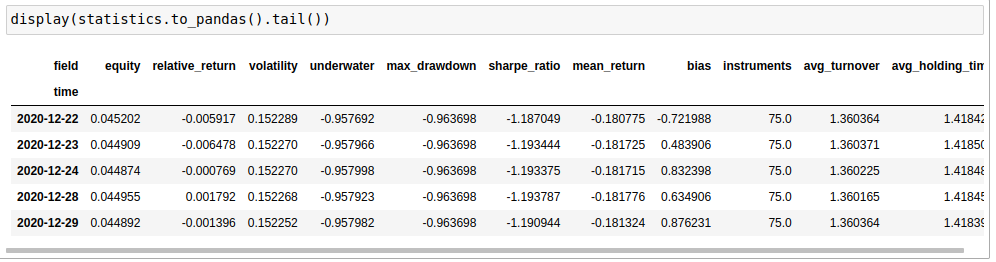Moreover we can produce a chart which shows the cumulative profits and losses:

```import qnt.graph as qngraph
performance = statistics.to_pandas()['equity']
qngraph.make_plot_filled(performance.index, performance, name='PnL (Equity)')
```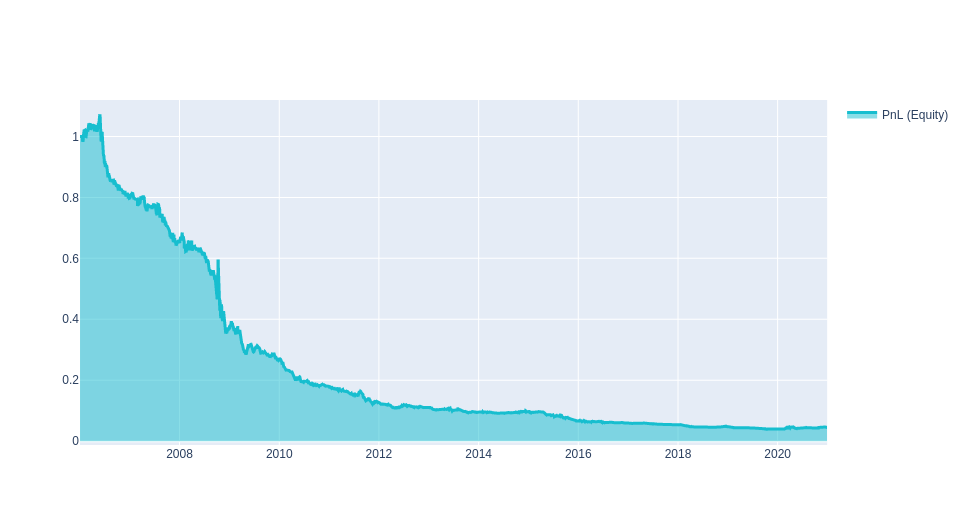For an overview of performance evaluation please consult our User Guide for Algorithm quality

4. Submit

Once you are satisfied with the quality of your algorithm you can submit it. The algorithm will be processed daily on our servers and it will accumulate a track record on live data. Each contest has a submission phase, during which you can submit code and replace it with new algos (you can have at most 50 running algorithms in your area), and a live phase, where submissions cannot be replaced and develop a track record.

The check function will show possible problems that your strategy has:

```output.check(weights, data, 'futures')
```

The first check is connected to the possible presence of missing values in your algorithm. With the previous call to the clean function, this problem is automatically solved.

The second check computes the In-Sample Sharpe ratio of your system. In this case, as the performance is negative, your submission would not be eligible for taking part to a contest. The In-Sample Sharpe ratio must be larger than 1.

The third check controls correlation with existing templates and with all systems submitted to previous contests.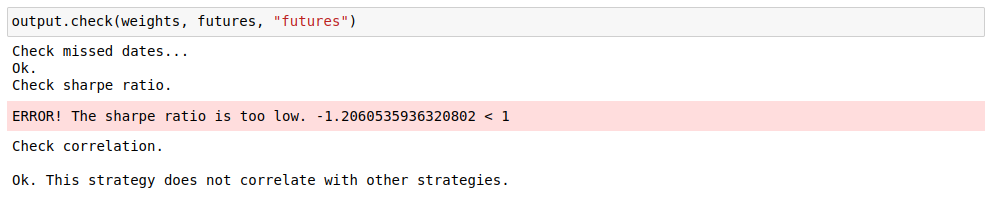If everything is ok, save the portfolio weights that the algorithm generates calling the write function:

```output.write(weights)
```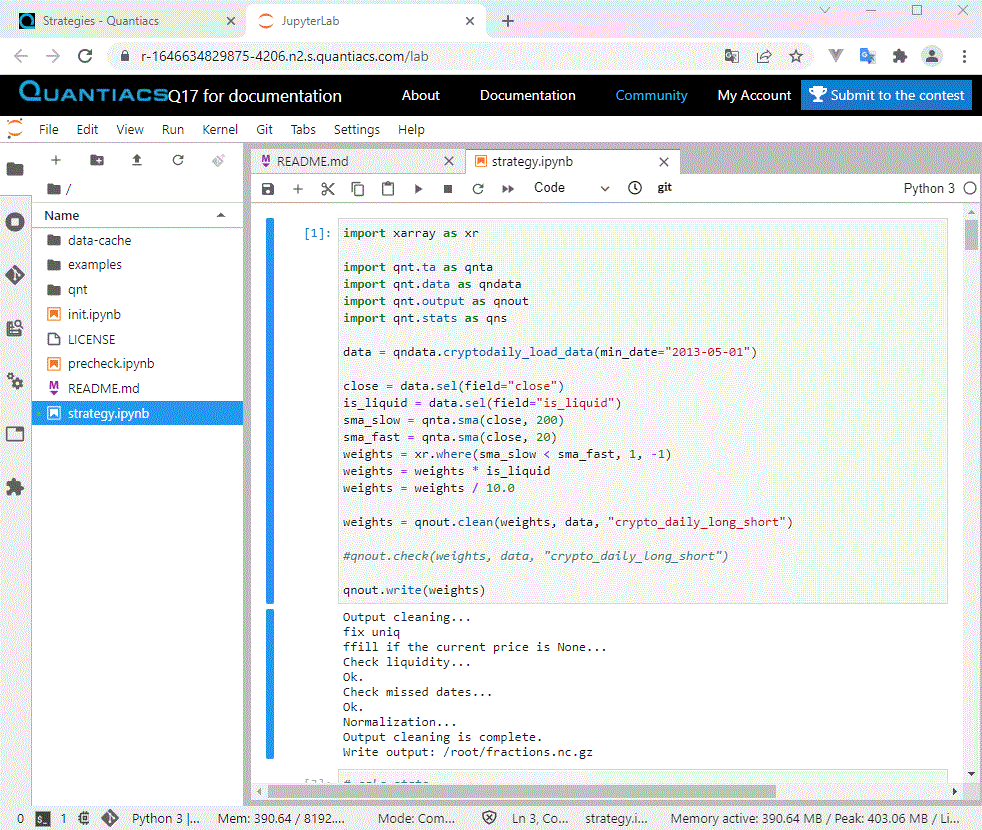To participate in the competition: